# Internediate Maths Solutions for Exercise 1(b) Functions

Inter Maths 1A text book solutions for exercise 1(b) Functions are given.

These solutions are very easy to understand. Please study the text book very well.

Observe the example problems and solutions. Try them.

Observe the given solutions and try them in your own method.

You can see the text book solutions for

Functions

Exercise 1(a)

Exercise 1(b)

Exercise 1(c)

Mathematical Induction

Exercise 2(a)

Matrices

Exercise 3(a)

Exercise 3(b)

Exercise 3(c)

Exercise 3(d)

Exercise 3(e)

Exercise 3(f)

Exercise 3(g)

Exercise 3(h)

Exercise 3(i)

Exercise 4(a)

Exercise 4(b)

Product of Vectors

Exercise 5(a)

Exercise 5(b)

Exercise 5(c)

Trigonometric Ratios upto Transformations

Exercise 6(a)

Exercise 6((b)

Exercise 6(c)

Exercise 6(d)

Exercise 6(e)

Exercise 6(f)

Trigonometric Equations

Exercise 7(a)

Inverse Trigonometric functions

Exercise 8(a)

Hyperbolic Functions

Exercise 9(a)

Properties of Triangles

Exercise 10(a)

Exercise 10(b)

Inter Maths Trigonometry text book solutions

Maths 1b

Locus

Exercise 1(a)

Transformations of axes

Exercise 2(a)

Three Dimensional Coordintes

Exercise 5(a)
Exercise 5(b)
The Plane

Exercise 7(a)
Lumits and continuity

Exercise 8(a)

Exercise 8(b)

M

# Inter Maths solutions for 1A Exercise 1(b) Functions

Exercise 1(b)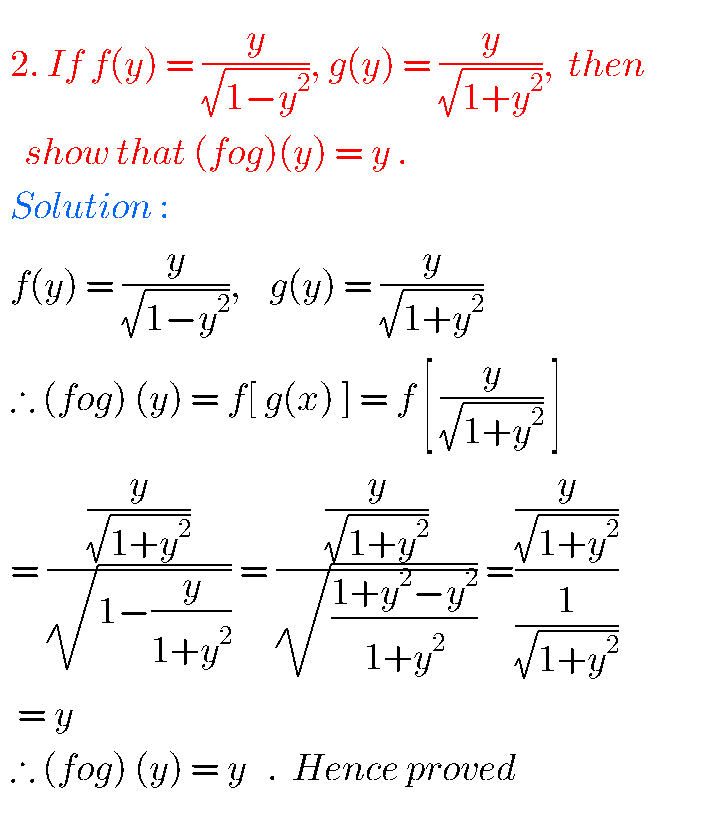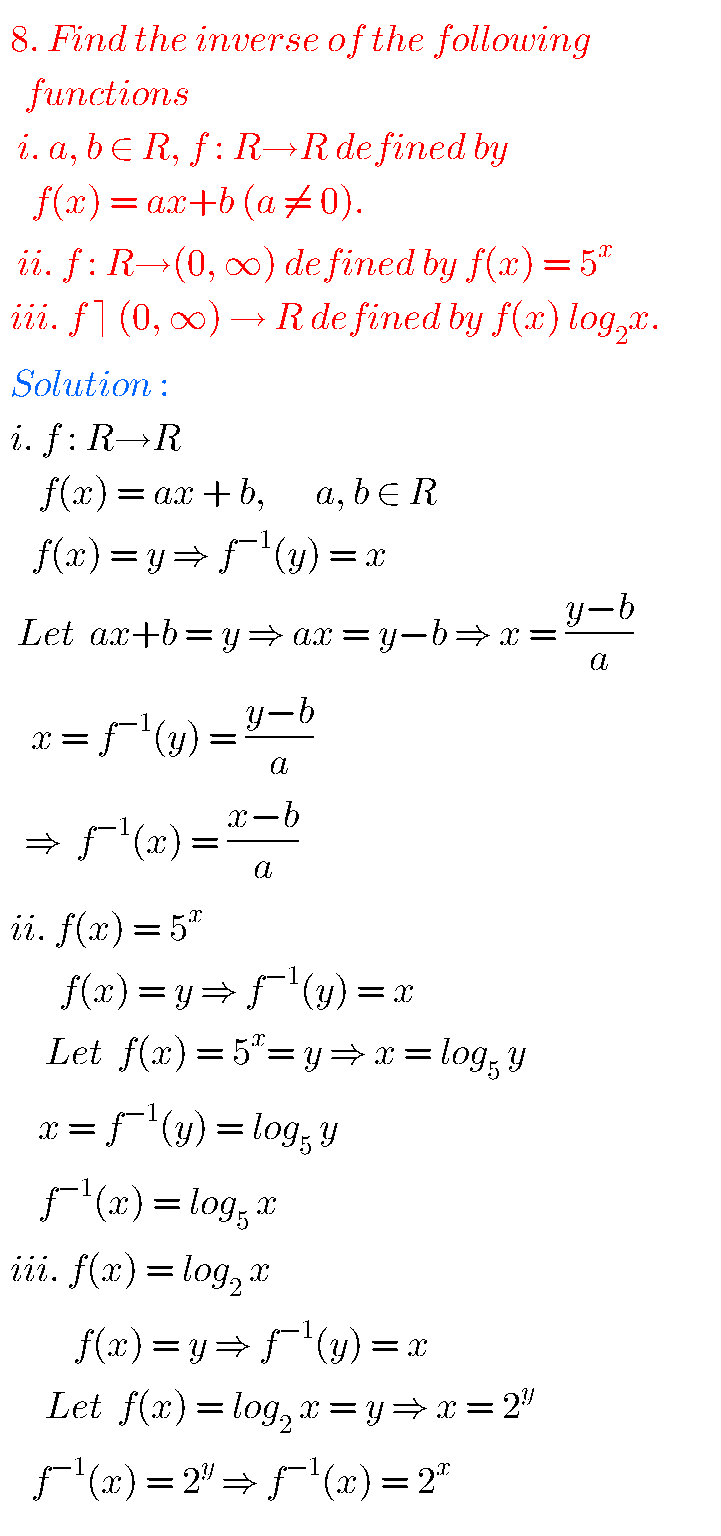## Functions solutions 1A Exercise 1(b)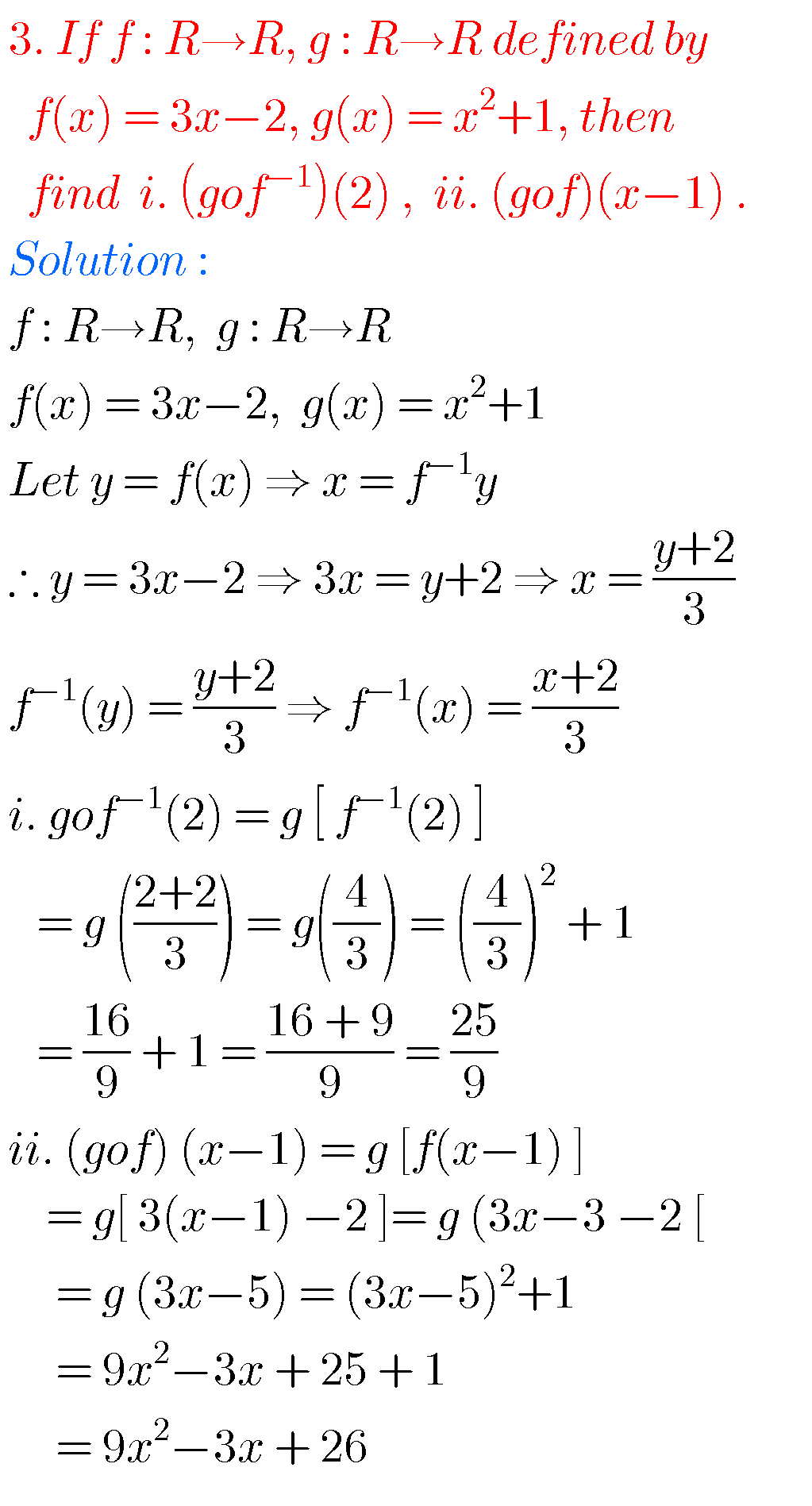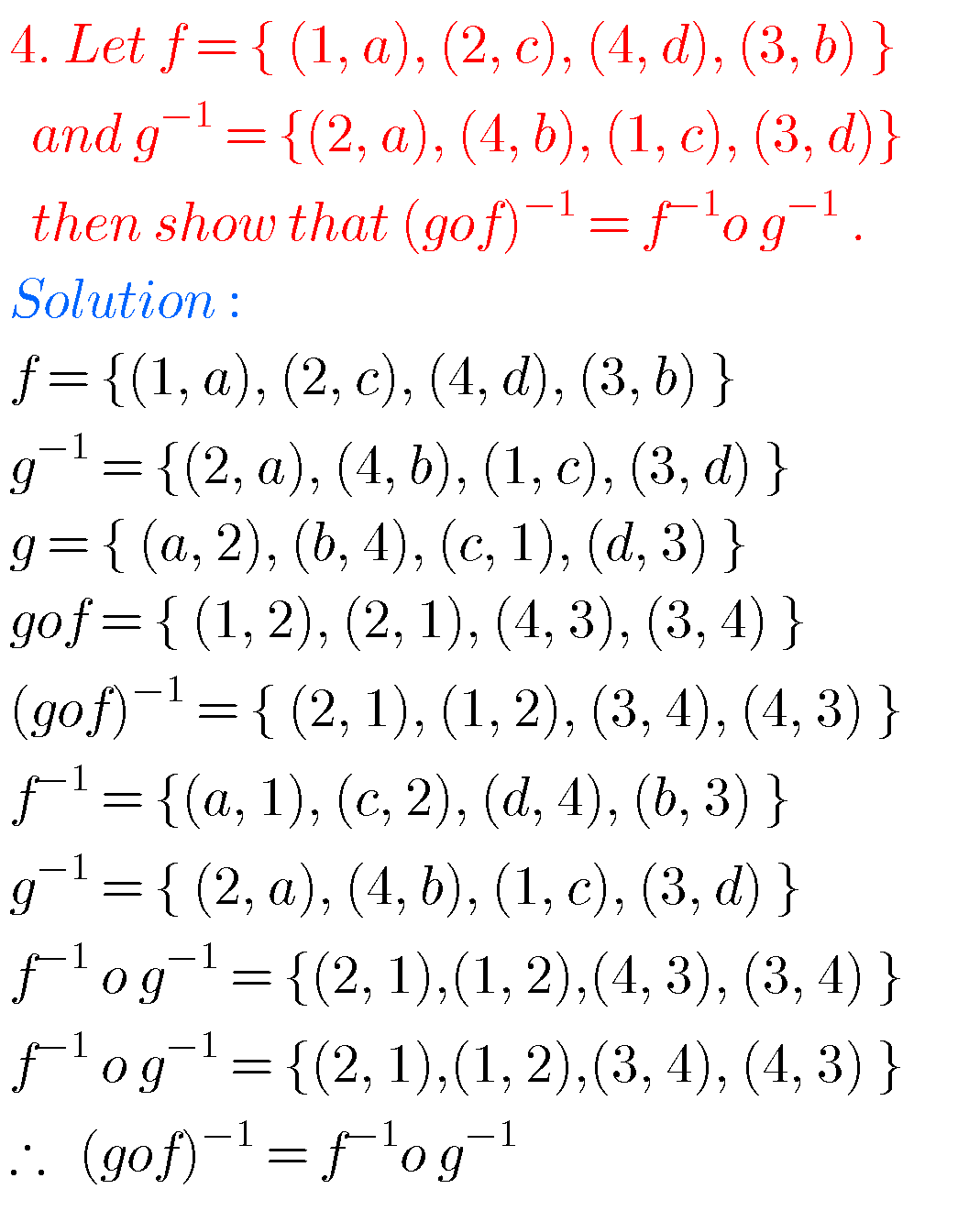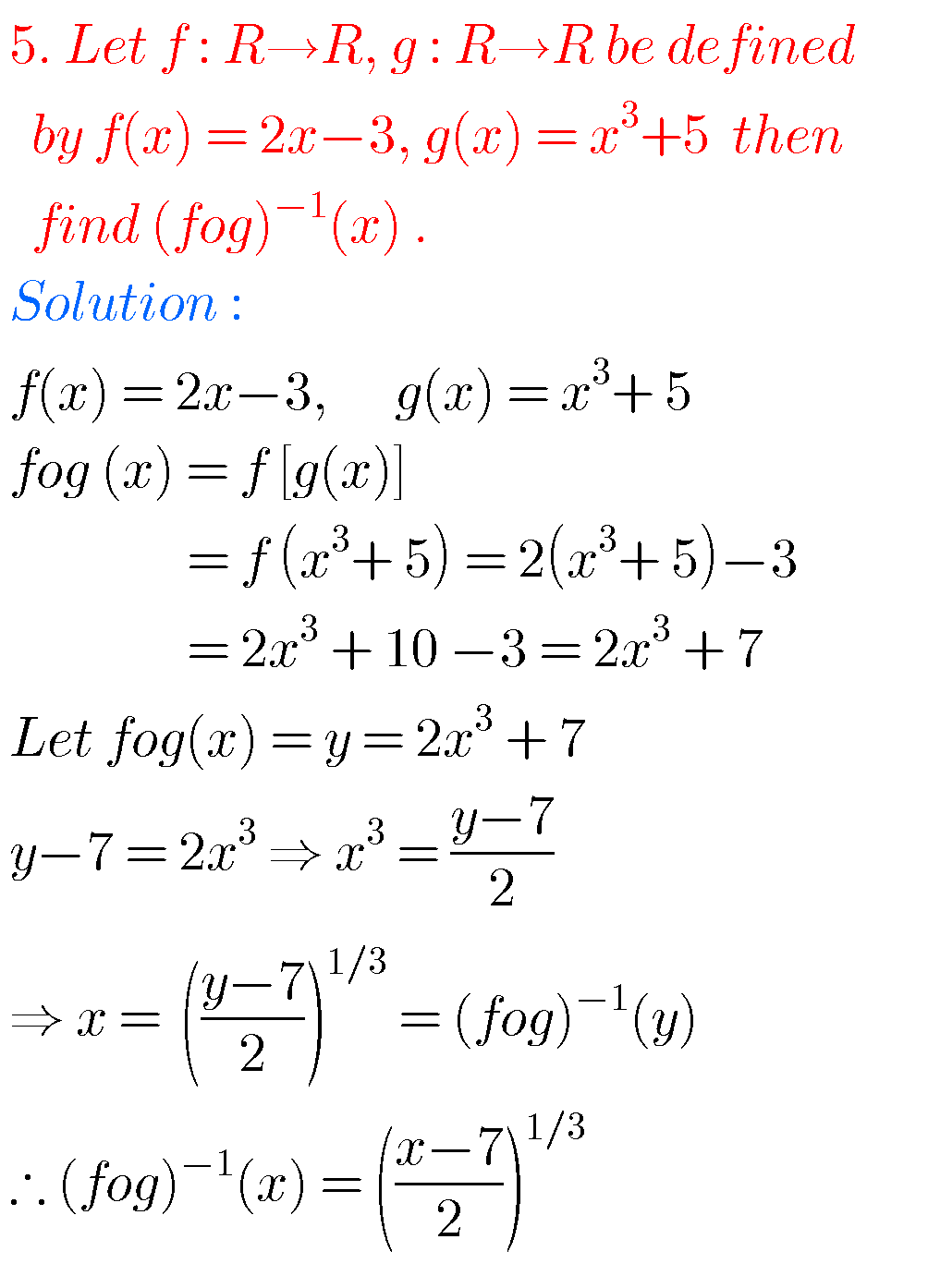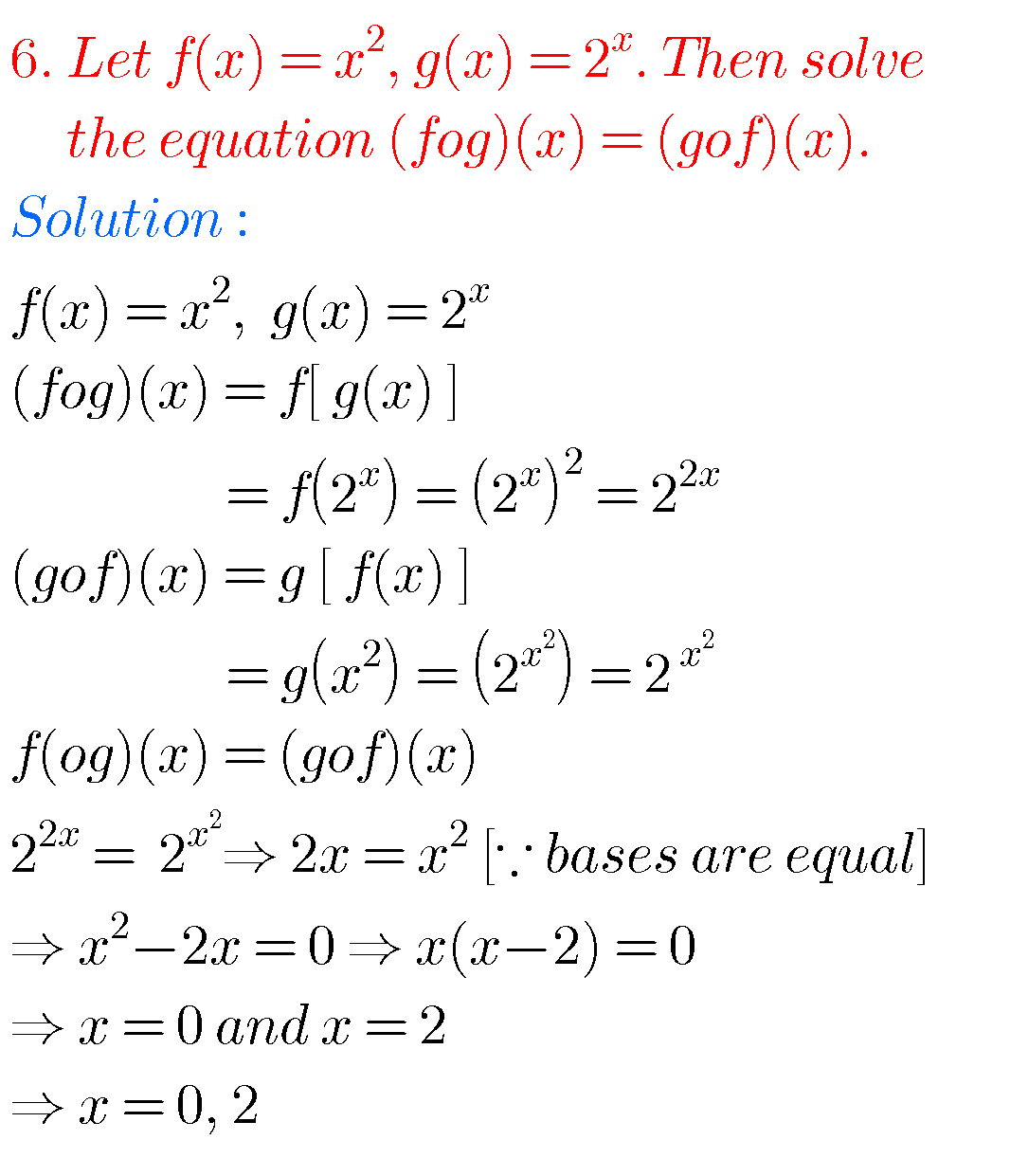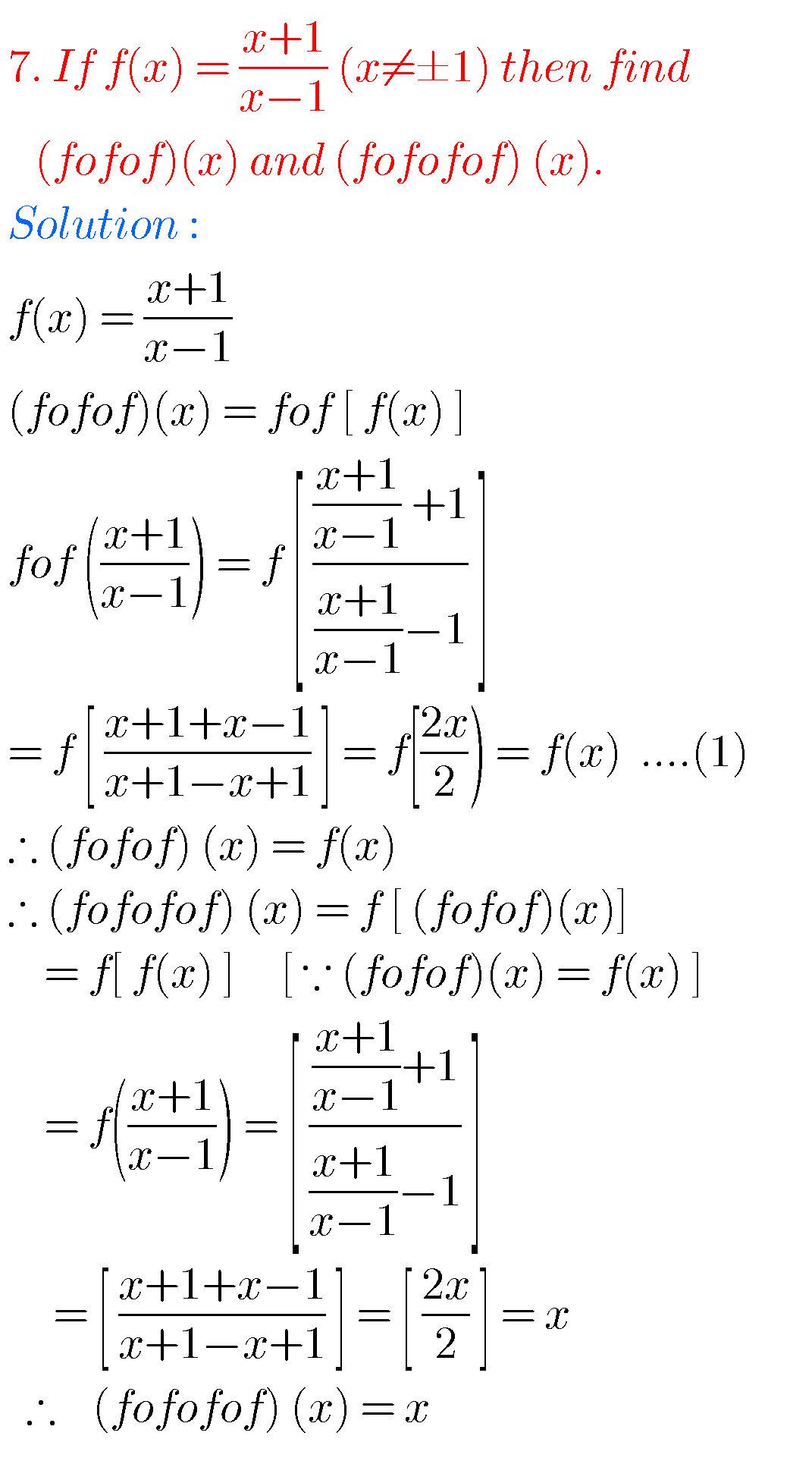Note : Observe the solutions and try them in your own method.

SSC Maths solutions

Inter 1a solutions

Inter 1b solutions

Inter 2a solutions